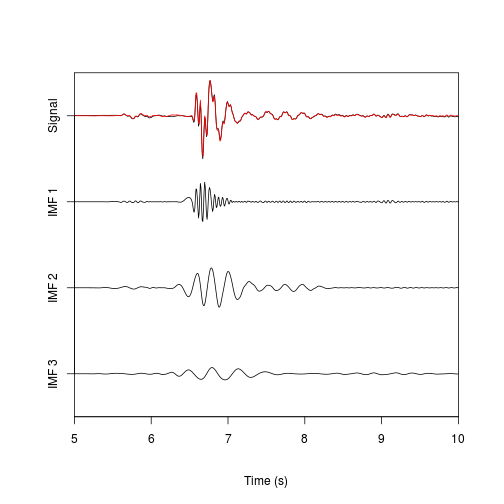## Electronic Supplement to The Hilbert-Huang Transform: A High Resolution Spectral Method for Nonlinear and Nonstationary Time Series

### by Daniel. C. Bowman and Jonathan M. Lees

This web page includes full color versions of the Hilbert and Fourier spectrogram figures as well as instructions on how to install and use the “hht” package in R. Data and code for all analyses performed in this paper are available for download here as well. Finally, we show how to generate a web page like this one using the “knitr” and “markdown” packages in R.

### Installing R and the hht package

R is an open-source programming language designed for scientific and statistical computation. The base R installation includes many functions for data analysis and display. Furthermore, over 4800 quality controlled, user contributed packages extend R's capabilities to many diverse areas. Below are instructions for installing and using the “hht” package, a series of functions designed for time series analysis using the Hilbert-Huang transform.

2. Once R is installed, open the R interpreter and download the “hht” package by typing:
install.packages("hht")


Linux users: start R as root if you want to make the package available to all users on the machine

Start the R interpreter and type (or copy and paste) the following:

library(hht, quietly = TRUE)  #Load hht package
data(PortFosterEvent)  #Use example data from hht package
emd.result <- Sig2IMF(sig, tt)  #Run EMD using default parameters
time.span <- c(5, 10)  #Time span to plot
imf.list <- 1:3  #IMFs to plot
os <- TRUE  #Show original signal in plot
res <- FALSE  #Do not show residual in plot
fit.line <- TRUE  #Plot a red line showing sum of displayed IMFs on original signal
PlotIMFs(emd.result, time.span = time.span, imf.list = imf.list, original.signal = os,
residue = res, fit.line = fit.line)### Generating Figure 1

The R code below will regenerate Figure 1.

library(hht, quietly = TRUE)  #Load hht package
dt <- 0.01
tt <- seq_len(200) * dt
sig1 <- sin(2 * pi * tt)
sig2 <- sin(2 * pi * tt) + 0.25

# Create analytic signals
asig1 <- HilbertTransform(sig1)
asig2 <- HilbertTransform(sig2)

# Get instantaneous frequency
fsig1 <- InstantaneousFrequency(asig1, tt)
fsig2 <- InstantaneousFrequency(asig2, tt)

plot(tt, fsig1, type = "l", ylim = c(0.5, 1.5), xlab = "Time (s)", ylab = "Instantaneous Frequency (Hz)",
lwd = 3)
lines(tt, fsig2, lty = 2, lwd = 3)### Generating Stokian wave example (Figures 2 through 4)

This example will generate individual gray scale and full color images for Figures 2 through 4.

1. Download the Stokian wave data and R script here and save it.
2. Unpack the tar.gz file.
3. Open the R interpreter and copy/paste code from stokes_wave.R to generate the data set.
4. Run each individual figure script to generate the Stokes wave figures.
5. Note that the EEMD method and may take several hours to complete. Therefore, we have included the script “parallel_eemd.R” to demonstrate how to run the EEMD on a cluster computer.

### Generating transient signal example (Figures 5 and 6)

This example will generate gray scale and full color images for Figures 5 and 6.

2. Open the R interpreter and copy/paste code from the scripts to generate the data set and Figures 5 and 6.

### Generating volcanic tremor example (Figures 7 through 10)

This example will generate gray scale and full color images files for Figures 7 through 10.

1. Download the tremor data and R script here and save it.
2. Unpack the tar.gz file.
3. Open the R interpreter and copy/paste code from the scripts to generate Figures 7 through 10.

Note: The EEMD section of this code will take weeks to run unless it is parallel processed. Therefore, we have included the script “parallel_eemd.R” to demonstrate how to run the EEMD on a cluster computer.

### Generating this web page

This web page was built using R also. You can generate a working copy of this web page by downloading the source file “SRL-D-13-00025-esupp.Rmd” here, downloading all links, saving the tar files in the same directory as “SRL-D-13-00025-esupp.Rmd” and the JPEG images in a directory called “color_figures”, and executing the following code:

install.packages("knitr")
install.packages("markdown")

library(hht)
library(knitr)
library(markdown)

knit2html("SRL-D-13-00025-esupp.Rmd")